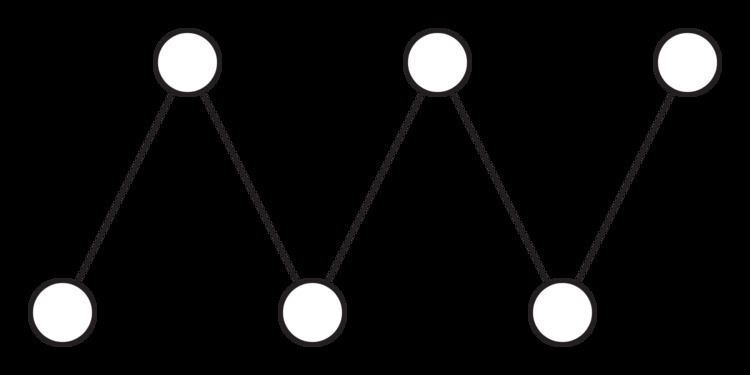# Fence (mathematics)

Updated onIn mathematics, a fence, also called a zigzag poset, is a partially ordered set in which the order relations form a path with alternating orientations:

a < b > c < d > e < f > h < i ...

or

a > b < c > d < e > f < h > i ...

A fence may be finite, or it may be formed by an infinite alternating sequence extending in both directions. The incidence posets of path graphs form examples of fences.

A linear extension of a fence is called an alternating permutation; André's problem of counting the number of different linear extensions has been studied since the 19th century. The solutions to this counting problem, the so-called Euler zigzag numbers or up/down numbers, are

1, 1, 2, 4, 10, 32, 122, 544, 2770, 15872, 101042 (sequence A001250 in the OEIS).

The number of antichains in a fence is a Fibonacci number; the distributive lattice with this many elements, generated from a fence via Birkhoff's representation theorem, has as its graph the Fibonacci cube.

A partially ordered set is series-parallel if and only if it does not have four elements forming a fence.

Several authors have also investigated the number of order-preserving maps from fences to themselves, or to fences of other sizes.

An up-down poset Q(a,b) is a generalization of a zigzag poset in which there are a downward orientations for every upward one and b total elements. For instance, Q(2,9) has the elements and relations

a > b > c < d > e > f < g > h > i.

In this notation, a fence is a partially ordered set of the form Q(1,n).

## Equivalent conditions

The following conditions are equivalent for a poset P:

1. P is a disjoint union of zigzag posets.
2. If abc in P, either a = b or b = c.
3. < < = , i.e. it is never the case that a < b and b < c, so that < is vacuously transitive.
4. P has dimension at most one (defined analogously to the Krull dimension of a commutative ring).
5. Every element of P is either maximal or minimal.
6. The slice category Pos/P is cartesian closed.

The prime ideals of a commutative ring R, ordered by inclusion, satisfy the equivalent conditions above if and only if R has Krull dimension at most one.

## References

Similar Topics
Virumbugiren
Dominic Reinold
Daniel Schildorfer
Topics